# Selection Sort in Python Programming

###### Sorting in Python

In this lesson, we will understand what is Selection Sort in Python Programming along with some examples.

## What is Selection Sort in Python?

A Selection Sort is a sorting technique used in python to sort elements of sequences like arrays and lists in ascending or descending order.

In this sorting technique, we will find the smallest element from the list and place it in the first position of the list. After that, we will take the second smallest number from the list and place it in the second position of the list in this way we will sort the entire list in ascending order.

To clearly understand the selection sorting technique, let's go through its algorithm step by step with an example.## Selection Sort Visualization

Suppose we have seven numbers stored in a list as shown below.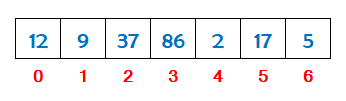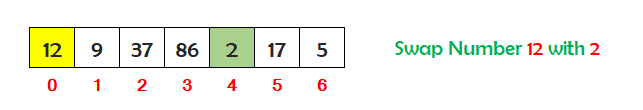Find the smallest number stored in the list between index 0 and 6. So the smallest number is 2. Check if the number at index 0 is greater than the smallest number at index 4. If yes, then swap the numbers.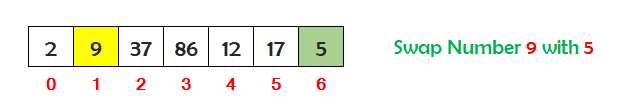Now find the second smallest number stored in the list between index 1 and 6. So the second smallest number is 5. Check if the number at index 1 is greater than the second smallest number at index 6. If yes, then swap the numbers.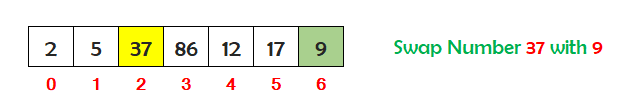Now find the third smallest number stored in the list between index 2 and 6. So the third smallest number is 9. Check if the number at index 2 is greater than the third smallest number at index 6. If yes, then swap the numbers.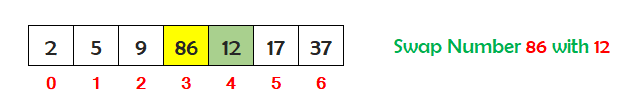Now find the fourth smallest number stored in the list between index 3 and 6. So the fourth smallest number is 12. Check if the number at index 3 is greater than the fourth smallest number at index 4. If yes, then swap the numbers.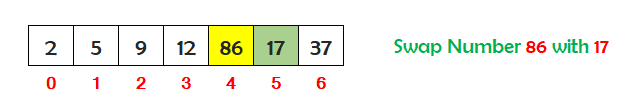Now find the fifth smallest number stored in the list between index 4 and 6. So the fifth smallest number is 17. Check if the number at index 4 is greater than the fifth smallest number at index 5. If yes, then swap the numbers.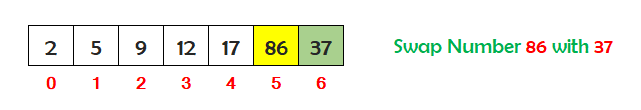Now find the sixth smallest number stored in the list between index 5 and 6. So the sixth smallest number is 37. Check if the number at index 5 is greater than the sixth smallest number at index 6. If yes, then swap the numbers.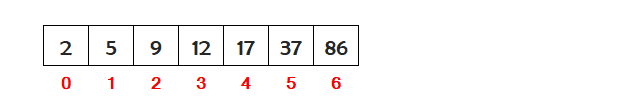So we can see that the entire list has sorted in ascending order.

## Algorithm for Selection Sort

An algorithm is the steps used to implement the selection sort in a python program.

Assume we have N numbers stored in a list. To implement the selection sort on N numbers, the steps are as follows.

• Define a list to store N numbers for selection sort. Suppose we have defined a list with the name num.
• Run an outer loop i from 0 to N-1 to repeat the process of selection sort.
• Store the value of i in a variable snp (smallest number position). For example snp=i.
• Run an inner loop j inside the body of the outer loop i for selection sort from i+1 to N.
• Inside the body of the inner loop j, check if the value at num[j] is smaller than the value at num[snp].
• If the value at num[j] is smaller than value at num[snp] then store the value of j to snp. For example snp=j.
• Outside the body of the inner loop j check if the value at num[i] is greater than that value at num[snp]. If yes, then swap the numbers.
• Now print the sorted list on the screen outside the body of the outer loop i.

## Python Program for Selection Sort

A Python program to sort a list of numbers in ascending order using the selection sort technique.

#### Selection Sort (List of Numbers in Ascending Order)

``````# define a list
num=[12,9,37,86,2,17,5]

print('List before Selection Sort')
for n in num:
print(n,end=' ')

# run an outer loop i from 0 to len(num)-1 to repeat the process of selection sort
for i in range(0,len(num)-1):

# smallest number position
snp=i

# run an inner loop j for selection sort from i+1 to len(num)
for j in range(i+1,len(num)):

# now check if the value at num[j] is smaller than value at num[snp]
if num[j]<num[snp]:
# if the value is smaller, then store the value of j to snp
snp=j

# outside the body of inner loop j check if num[i]>num[snp]. If yes then swap the numbers
if num[i]>num[snp]:
t=num[i]
num[i]=num[snp]
num[snp]=t

# print the sorted list
print('\n\nList after Selection Sort')
for n in num:
print(n,end=' ')``````

#### Output

```List before Selection Sort
12 9 37 86 2 17 5

List after Selection Sort
2 5 9 12 17 37 86```

A Python program to sort a list of numbers in descending order using the selection sort technique.

#### Selection Sort (List of Numbers in Descending Order)

``````# define a list
num=[12,9,37,86,2,17,5]

print('List before Selection Sort')
for n in num:
print(n,end=' ')

# run an outer loop i from 0 to len(num)-1 to repeat the process of selection sort
for i in range(0,len(num)-1):

# largest number position
lnp=i

# run an inner loop j for selection sort from i+1 to len(num)
for j in range(i+1,len(num)):

# now check if the value at num[j] is greater than value at num[lnp]
if num[j]>num[lnp]:
# if the value is greater, then store the value of j to lnp
lnp=j

# outside the body of inner loop j check if num[i]<num[snp]. If yes then swap the numbers
if num[i]<num[lnp]:
t=num[i]
num[i]=num[lnp]
num[lnp]=t

# print the sorted list
print('\n\nList after Selection Sort')
for n in num:
print(n,end=' ')``````

#### Output

```List before Selection Sort
12 9 37 86 2 17 5

List after Selection Sort
86 37 17 12 9 5 2```

A Python program to sort a list of strings in ascending order using the selection sort technique.

#### Selection Sort (List of Strings in Ascending Order)

``````# define a list
num=['Squirrel','Dog','Panda','Lion','Bear','Tiger','Rabbit']

print('List before Selection Sort')
for n in num:
print(n,end='  ')

# run an outer loop i from 0 to len(num)-1 to repeat the process of selection sort
for i in range(0,len(num)-1):

# smallest string position
snp=i

# run an inner loop j for selection sort from i+1 to len(num)
for j in range(i+1,len(num)):

# now check if the value at num[j] is smaller than value at num[snp]
if num[j]<num[snp]:
# if the value is smaller then store the value of j to snp
snp=j

# outside the body of inner loop j check if num[i]>num[snp]. If yes then swap the strings
if num[i]>num[snp]:
t=num[i]
num[i]=num[snp]
num[snp]=t

# print the sorted list
print('\n\nList after Selection Sort')
for n in num:
print(n,end='  ')``````

#### Output

```List before Selection Sort
Squirrel  Dog  Panda  Lion  Bear  Tiger  Rabbit

List after Selection Sort
Bear  Dog  Lion  Panda  Rabbit  Squirrel  Tiger```

## Selection Sort Program Explanation

The explanation of the above program (List of Numbers in Ascending Order) is given below in details.

#### Explanation

```List before Selection Sort
12 9 37 86 2 17 5

The outer loop i starts running from 0 to 5

When i = 0
snp = 0

The inner loop j starts running from 1 to 6

When j = 1
List is : 12 9 37 86 2 17 5    Check if 9<12   Yes 9<12 then store the value of j to snp
snp = 1

When j = 2
List is : 12 9 37 86 2 17 5    Check if 37<9   No

When j = 3
List is : 12 9 37 86 2 17 5    Check if 86<9   No

When j = 4
List is : 12 9 37 86 2 17 5    Check if 2<9   Yes 2<9 then store the value of j to snp
snp = 4

When j = 5
List is : 12 9 37 86 2 17 5    Check if 17<2   No

When j = 6
List is : 12 9 37 86 2 17 5    Check if 5<2   No

The inner loop ends. Now check if 12>2. If yes then swap the numbers
List is : 2 9 37 86 12 17 5

When i = 1
snp = 1

The inner loop j starts running from 2 to 6

When j = 2
List is : 2 9 37 86 12 17 5    Check if 37<9   No

When j = 3
List is : 2 9 37 86 12 17 5    Check if 86<9   No

When j = 4
List is : 2 9 37 86 12 17 5    Check if 12<9   No

When j = 5
List is : 2 9 37 86 12 17 5    Check if 17<9   No

When j = 6
List is : 2 9 37 86 12 17 5    Check if 5<9   Yes 5<9 then store the value of j to snp
snp = 6

The inner loop ends. Now check if 9>5. If yes then swap the numbers
List is : 2 5 37 86 12 17 9

When i = 2
snp = 2

The inner loop j starts running from 3 to 6

When j = 3
List is : 2 5 37 86 12 17 9    Check if 86<37   No

When j = 4
List is : 2 5 37 86 12 17 9    Check if 12<37   Yes 12<37 then store the value of j to snp
snp = 4

When j = 5
List is : 2 5 37 86 12 17 9    Check if 17<12   No

When j = 6
List is : 2 5 37 86 12 17 9    Check if 9<12   Yes 9<12 then store the value of j to snp
snp = 6

The inner loop ends. Now check if 37>9. If yes then swap the numbers
List is : 2 5 9 86 12 17 37

When i = 3
snp = 3

The inner loop j starts running from 4 to 6

When j = 4
List is : 2 5 9 86 12 17 37    Check if 12<86   Yes 12<86 then store the value of j to snp
snp = 4

When j = 5
List is : 2 5 9 86 12 17 37    Check if 17<12   No

When j = 6
List is : 2 5 9 86 12 17 37    Check if 37<12   No

The inner loop ends. Now check if 86>12. If yes then swap the numbers
List is : 2 5 9 12 86 17 37

When i = 4
snp = 4

The inner loop j starts running from 5 to 6

When j = 5
List is : 2 5 9 12 86 17 37    Check if 17<86   Yes 17<86 then store the value of j to snp
snp = 5

When j = 6
List is : 2 5 9 12 86 17 37    Check if 37<17   No

The inner loop ends. Now check if 86>17. If yes then swap the numbers
List is : 2 5 9 12 17 86 37

When i = 5
snp = 5

The inner loop j starts running from 6 to 6

When j = 6
List is : 2 5 9 12 17 86 37    Check if 37<86   Yes 37<86 then store the value of j to snp
snp = 6

The inner loop ends. Now check if 86>37. If yes then swap the numbers
List is : 2 5 9 12 17 37 86

The outer loop ends

List after Selection Sort
2 5 9 12 17 37 86```